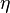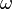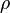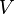## Tuesday, May 19, 2015

### Measuring the viscosity of the electron fluid in a metal

Previously I posted about the theoretical issue of the viscosity of the electron fluid in strongly correlated metals. This interest is partly motivated by claims from string theory techniques [AdS-CFT] that there is a universal lower bound for the viscosity.  A recent experimental paper estimated the viscosity in the cuprates by an indirect method from ARPES data.

I only became aware recently that there is a somewhat direct way to measure the viscosity of the electron fluid in a metallic crystal. This has a long history going back to Mason and Pippard who in 1955 related the viscosity to the attenuation of sound. A more sophisticated and general theory was developed by Kahn and Allen.

The connection between shear viscosity and ultrasound attenuation can be loosely motivated as follows. In a viscous fluid the attenuation of a shear wave is given by Stokes law

where$\eta$ is the shear viscosity of the fluid,$\omega$ is the sound's frequency$\rho$ is the fluid density, and$V$ is the speed of sound in the medium.

This equation has been used to determine the shear viscosity as a function of temperature for helium three [a correlated neutral fermion fluid]. Extensive experimental data is reviewed here.

In a metal, provided the wavelength of sound is much larger than the electronic mean free path, then one is in the hydrodynamic limit, and the attenuation is given by a similar expression to that above (with appropriate indices for crystal axes), with$\rho$ the solid density (not the electron fluid).

One can show from the Boltzmann equation that in a simple free electron model that the electronic viscosity is proportional to the scattering time, just like the conductivity. Hence, the ultrasound attenuation should scale with the conductivity.

Indirect evidence for this idea is from the data below that shows the temperature dependence of ultrasound attenuation of aluminium (taken from here).

In clean metals, such as for the data shown above, the attenuation [and viscosity] becomes very large at low temperatures, making it easier to measure.
Also, for high frequency ultrasound, one can reach the "quantum regime" where the mean free path becomes comparable to the sound wavelength. Pippard worked out a general theory describing the crossover from the hydrodynamic regime to this quantum regime.

In bad metals could one experimentally see the small viscosity, of the order of n hbar [where n is the density]? First, the small mean free path, characteristic of bad metals, means one will always be in the hydrodynamic regime. However, the small viscosity means that the sound attenuation due to the electron fluid will be small and possibly dominated by other sources of attenuation such as crystal dislocations. A rough estimate for an electron viscosity of order of n hbar and a sound frequency of 1 GHz gives an attenuation of less than 0.1 cm-1, of the order of typical sensitivity, such as in these measurements for heavy fermion compounds.